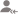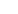true

Take Class 10 Tuition from the Best Tutors

•Affordable fees
•1-1 or Group class
•Flexible Timings
•Verified Tutors

Search in# Learn UNIT IV: Geometry with Free Lessons & Tips

Post a Lesson

All

All

Lessons

Discussion

1. Draw an isosceles triangle ABC in which AB = AC = 6 cm and BC = 5 cm. Construct a triangle PQR similar... read more
1. Draw an isosceles triangle ABC in which AB = AC = 6 cm and BC = 5 cm. Construct a triangle PQR similar to ∆ ABC in which PQ = 8 cm. Also, justify the construction. read less

2. Let ABC be a right triangle in which AB = 6 cm, BC = 8 cm and B = 90 . BD is perpendicular to B... read more
2. Let ABC be a right triangle in which AB = 6 cm, BC = 8 cm and ∠ B = 90°. BD is perpendicular to B on AC. The circle through B, C, D is drawn. Construct the tangents from A to this circle. read less

4. Draw a triangle ABC with side BC = 6 cm, AB = 5 cm and ABC = 60 . Then construct a triangle whose... read more
4. Draw a triangle ABC with side BC = 6 cm, AB = 5 cm and ∠ABC = 60°. Then construct a triangle whose sides are 3/4 of the corresponding sides of the triangle ABC. read less

Take Class 10 Tuition from the Best Tutors

•Affordable fees
•Flexible Timings
•Choose between 1-1 and Group class
•Verified Tutors

5. Draw a triangle PQR with side QR = 7 cm, angle Q = 45 degrees and Angle A = 105 degrees. After that,... read more
5. Draw a triangle PQR with side QR = 7 cm, angle Q = 45 degrees and Angle A = 105 degrees. After that, Construct a triangle whose sides are 3/4 of the corresponding sides of triangle ABC. read less

6. Use a bangle to draw a circle and take a point outside the circle. Now construct a pair of tangents... read more
6. Use a bangle to draw a circle and take a point outside the circle. Now construct a pair of tangents from A to this circle. read less

7. Draw a line segment of length 7.6 cm and divide it in the ratio 5:8. Measure the two parts.

Take Class 10 Tuition from the Best Tutors

•Affordable fees
•Flexible Timings
•Choose between 1-1 and Group class
•Verified Tutors

8. Draw a pair of tangents to a circle of radius 5 cm which is inclined to each other at an angle of 60 degrees.

9. Draw a triangle with sides 5 cm, 6 cm and 7 cm. Draw another triangle whose sides are 4/5 of the corresponding... read more
9. Draw a triangle with sides 5 cm, 6 cm and 7 cm. Draw another triangle whose sides are 4/5 of the corresponding sides of the first triangle. read less

10. Construct a ABC in which AB = 6 cm, A = 30 and B = 60 . Construct another AB C similar to ... read more
10. Construct a ΔABC in which AB = 6 cm, ∠A = 30° and ∠B = 60°. Construct another ΔAB’C’ similar to ΔABC with base AB’ = 8 cm. read less

Take Class 10 Tuition from the Best Tutors

•Affordable fees
•Flexible Timings
•Choose between 1-1 and Group class
•Verified Tutors

1. How many tangents can a circle have?Rajn Andini Tiwari

Sophomores NIT Bhopal with 2 years of tutoring experience

A circle can have infinite tangents.
Dislike Bookmark

UrbanPro.com helps you to connect with the best Class 10 Tuition in India. Post Your Requirement today and get connected.

Overview

Questions 30

Total Shares3 Followers

## Top Contributors

Connect with Expert Tutors & Institutes for UNIT IV: Geometry

## Class 10 Tuition in:

x

X

### Looking for Class 10 Tuition Classes?

The best tutors for Class 10 Tuition Classes are on UrbanPro

• Select the best Tutor
• Book & Attend a Free Demo
• Pay and start Learning### Take Class 10 Tuition with the Best Tutors

The best Tutors for Class 10 Tuition Classes are on UrbanProUrbanPro.com is India's largest network of most trusted tutors and institutes. Over 55 lakh students rely on UrbanPro.com, to fulfill their learning requirements across 1,000+ categories. Using UrbanPro.com, parents, and students can compare multiple Tutors and Institutes and choose the one that best suits their requirements. More than 7.5 lakh verified Tutors and Institutes are helping millions of students every day and growing their tutoring business on UrbanPro.com. Whether you are looking for a tutor to learn mathematics, a German language trainer to brush up your German language skills or an institute to upgrade your IT skills, we have got the best selection of Tutors and Training Institutes for you. Read more